# How to Create Pivot Table Calculated FieldsAlex Williams

Pivot tables are one of the most useful tools in Excel. In fact, it is the first thing you’ll find on the Insert tab of Excel.

At a basic level, pivot tables allow you to present information in a way that is both quick and intuitive to understand. If you’re new to pivot tables, you can refer to our guide on how to create a pivot table in five easy steps to get started.

One of the benefits of pivot tables is that you can interactively change how the data is presented for a specific need. With that in mind, let’s jump straight into pivot table calculated fields.

Use this free calculated field Excel file to practice along with the tutorial.

## What is a calculated field?

An Excel pivot table calculated field essentially functions as an individual field where you can set a custom calculation.

This calculation field sums the information in other fields and then does the specific calculation you want on it. As such, it is important to know how calculated fields work so that you can customize your pivot table calculated items.

## How to add a calculated field

### Simple calculated field

So how do you create a calculated field in pivot tables? Well, let’s take this pivot table of different baked goods, their unit prices, and their total sums.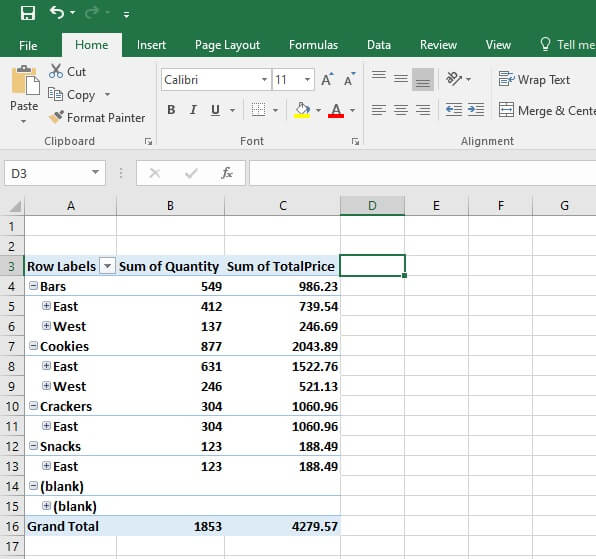At this point, highlight your pivot table, which should create a new little tab at the top which says ‘pivot table’ with two tabs underneath it. You’ll want to click on Analyze and then Field, Items & Sets. Then finally select Calculated field.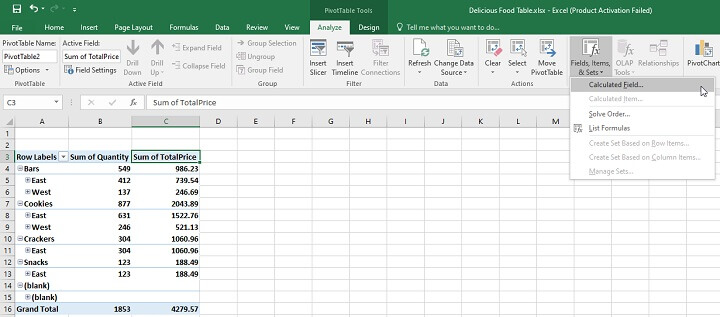As you’ll see, a new window will come up for you to create a calculated field.

For this example, we’ll calculate a percentage of tax on the price of each item, which will be 14%.

To do this, fill out the Name area with Food Tax. Then we need to create the actual calculation, which will go into the Formula area.

For this, we’ll use the Sum of Total Price column, which you will see listed as ‘TotalPrice’ in the fields section below. Just double click that to put that as the column we want to add, then append it with ‘ * 0.14 ‘, which means to multiply it by 0.14 or 14%.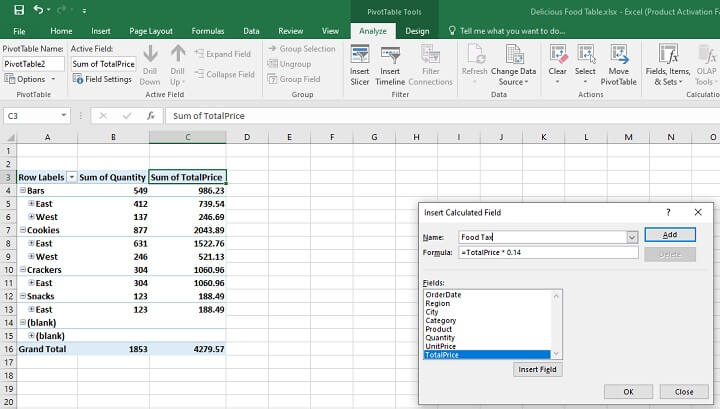Once you’re done, click Add, which will add it to the fields list, as well as the pivot table itself once you click Ok.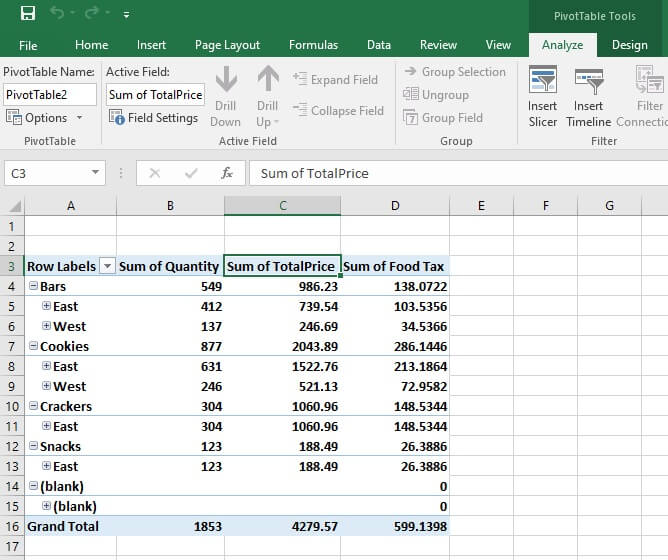And there you see what the tax would be for each item in their respective row.

### Complex calculated field

Complex calculated fields are exactly the same as simple calculated fields, with the main difference being that the formula you plug in might be more complicated. For example, instead of calculating only a 14% tax on the food items in the example above, you could also add a sales tax on top of that.

This can certainly be helpful with places that might not have the same calculations across the board, so being able to add a bit more complexity to the formula is great.

## How to modify a calculated field

### How to remove a calculated field

If you would like to temporarily remove a calculated field, just right-click on the field and then choose the Remove option, which is ‘Remove "Sum of Food Tax’ in this case.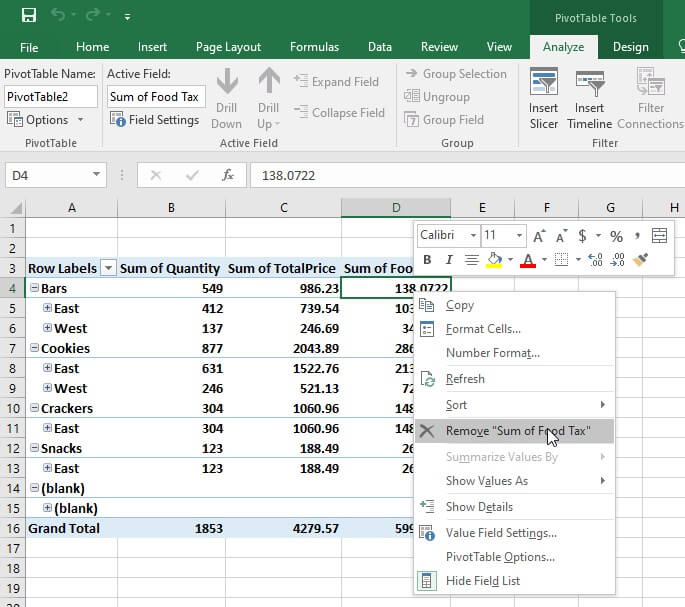On the other hand, if you want to remove a field permanently, head back to the Insert Calculated Field box by going to Analyze > Field, Items & Sets

Once there, in the Name area you’ll find a drop-down list of the calculated fields. Select the one you don’t want and then click Delete.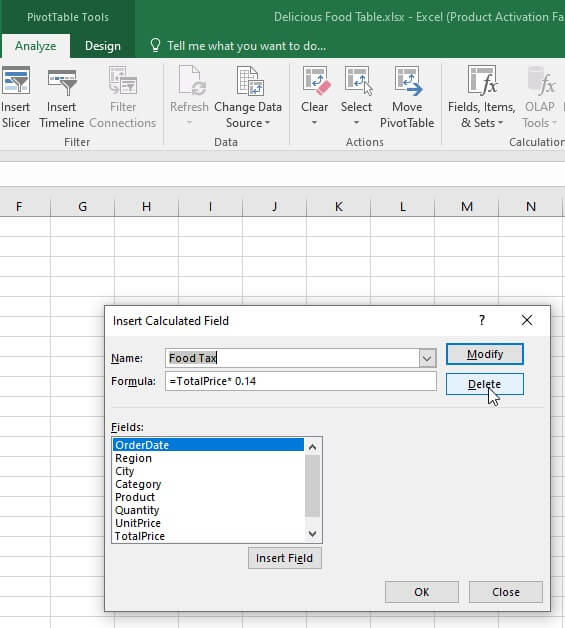## How to get a list of calculated field formulas

Getting a list of calculated formulas in Excel is relatively easy. Just highlight any field on the pivot table, go to the top ribbon where it says Analyze and then go to Fields, Items & Sets and click on List Formulas.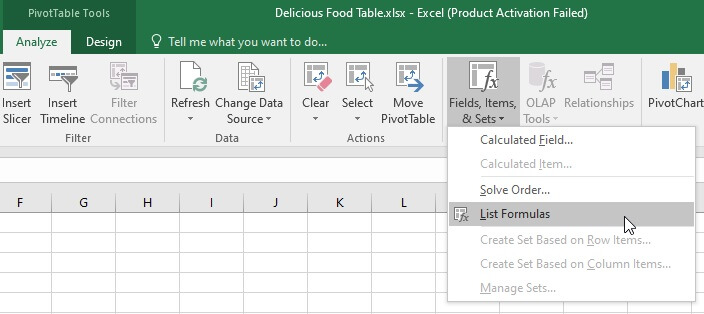After that, a new sheet will open up with any formulas used in your calculated field.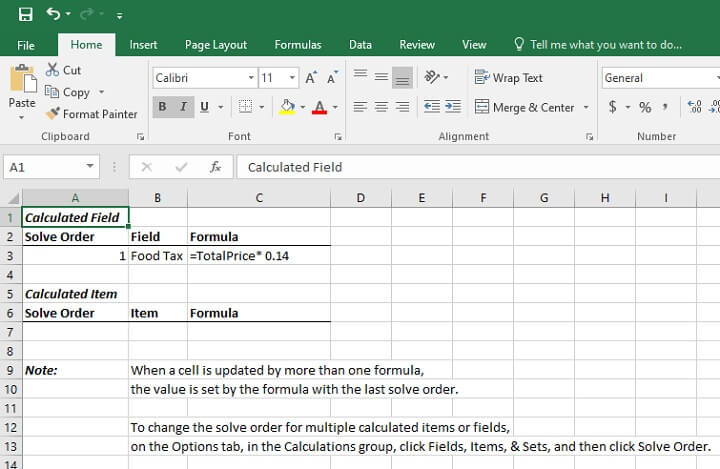## Challenges with calculated fields

Normally you would expect to see a sum of the calculated amounts when it comes to the pivot table. Excel, on the other hand, calculates fields using the same calculation for both the subtotals and grand totals rows, rather than showing a sum.

## Differences between Office versions

Aside from a few UI design choices, the general layout and naming of all the steps are exactly the same across Office versions. This is great if you’re switching from an older version to a new one.

## When not to use a pivot table

Did you know Excel has a limitation of 1,048,576 rows of data? Most users won’t even come close to this number, but such cases exist. If your work requires you to manage a giant table, that for example has two million rows, pivot tables won’t get the job done all by themselves.

Thus, in 2010, Excel introduced an add-in called PowerPivot. It allows you not only to surpass this limit but also to produce more efficient workbooks than the ones created by regular pivot tables.

However, PowerPivot is a different topic — if you are interested, check out our guide on how to use, install, and set up PowerPivot.

## Final words

We’ve talked about the basic pivot table calculated field functions and use cases. If you’re interested in more, our guide to advanced pivot table techniques is sure to help you.

If you would truly like to master pivot tables, try our Pivot Tables - From Novice to Ninja course. It is designed to help you cover the fundamentals that you might’ve missed or need a refresher on, and show you the endless possibilities pivot tables can offer.